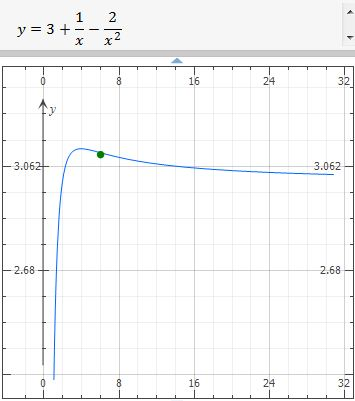# Consider a differentiable function f having a domain of all positive real numbers, and for which...

## Question:

Consider a differentiable function f having a domain of all positive real numbers, and for which it is known that {eq}f'(x)= (4 - x)x^{-3} {/eq} for x > 0.

a. Find all intervals on which the graph of f is concave down. Justify answer.

b. Given that f(1)= 2 determine f(x).

## Concavity intervals and inflection point

If we have the first derivative, and we need the concave down intervals, we find the second derivative and make a second derivative test, remember: a Function with one independent variable has inflection point where its second derivative is zero, also if the second derivative is positive the function is concave up and if the second derivative is negative, the function is concave down.

The first derivative is: {eq}\displaystyle \ f'(x)= (4 - x)x^{-3} \\ {/eq}

The second derivative is: {eq}\displaystyle \ f''(x)= 2\,{x}^{-3}-12\,{x}^{-4} \\ \displaystyle \ f''(x) = 2\,{\frac {x-6}{{x}^{4}}} \\ {/eq}

Inflection point at {eq}\displaystyle \ f''(x) =0 \; \Rightarrow \; 0= 2\,{\frac {x-6}{{x}^{4}}} \; \Leftrightarrow \; x= 6 \\ {/eq} so, the inflection point is: {eq}(6 , f(6)) {/eq}

Second derivative test:

{eq}\begin{array} \; \text{Interval} \; & { 0 < x < 6 } & { 6 < x < \infty } \\ \hline Test \space{} value & { x = 2 } & { x = 9 } \\ Test & { f''( 2 ) = - < 0 } & { f''( 9 ) = + > 0 } \\ Conclusion & { concave \; downward } & { concave \; upward } \\ \end{array} \\ \therefore \boxed { \text{ concave upward interval is} \; \ ( 6 , \infty )} \\ \therefore \boxed { \text{ concave downward interval is} \; \ ( 0 , 6 )} \\ {/eq}

a. Therefore, the interval on which the graph of f is concave down is {eq}( 0 , 6 ) \\ {/eq}

b. Given that f(1)= 2

{eq}\ f(x) {/eq} is

{eq}\displaystyle \int \ f'(x) \;dx = \int (4 - x)x^{-3} \; dx \\ \displaystyle \ f(x) = {x}^{-1}-2\,{x}^{-2} + C \\ {/eq}

Now, with the given point: {eq}(1,2 ) \\ \displaystyle 2= {(1)}^{-1}-2\,{(1)}^{-2}+C \; \Rightarrow \; C= 3 \\ {/eq}

Therefore, the function is:

{eq}\displaystyle \ f(x) = {x}^{-1}-2\,{x}^{-2}+3 \\ {/eq}

Graph